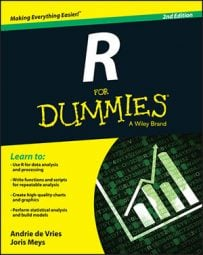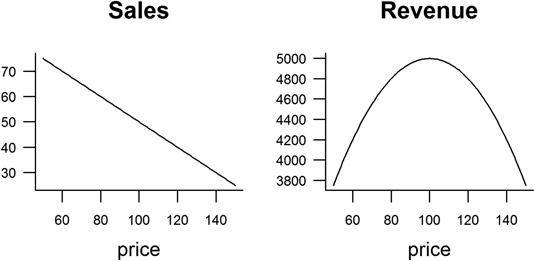##### R For DummiesThe spreadsheet is probably one of the most widely used PC applications — and for good reason: Spreadsheets make it very easy to perform calculations and other operations on tabular data. But spreadsheets pose some risks as well: They’re easy to corrupt and very difficult to debug. The good news is, you can use R to do many of the same things you used to do in spreadsheets.

## Adding row and column totals

One task that you may frequently do in a spreadsheet is calculating row or column totals. The easiest way to do this is to use the functions rowSums() and colSums(). Similarly, use rowMeans() and colMeans() to calculate means.

Try it on the built-in dataset iris. First, remove the fifth column, because it contains text that describes the species of iris:

`> iris.num <- iris[, -5]`

Then calculate the sum and mean for each column:

```> colSums(iris.num)
> colMeans(iris.num)```

These two functions are very convenient, but you may want to calculate some other statistic for each column or row. There’s an easy way of traversing rows or columns of an array or data frame: the apply() function. For example, getting the minimum of a column is the same as applying a min() function to the second dimension of your data:

```> apply(iris.num, 2, min)
> apply(iris.num, 2, max)```

## Formatting numbers

You can use format() to turn your numbers into pretty text, ready for printing. This function takes a number of arguments to control the format of your result. Here are a few:

• trim: A logical value. If FALSE, it adds spaces to right-justify the result. If TRUE, it suppresses the leading spaces.

• digits: How many significant digits of numeric values to show.

• nsmall: The minimum number of digits after the decimal point.

In addition, you control the format of the decimal point with decimal.mark, the mark between intervals before the decimal point with big.mark, and the mark between intervals after the decimal point with small.mark.

For example, you can print the number 12345.6789 with a comma as decimal point, spaces as the big mark, and dots as the small mark:

```> format(12345.6789, digits = 9, decimal.mark = ",",
+    big.mark = " ", small.mark = ".", small.interval = 3)
 "12 345,678.9"```

As a more practical example, to calculate the means of some columns in mtcars and then print the results with two digits after the decimal point, use the following:

```> x <- colMeans(mtcars[, 1:4])
> format(x, digits = 2, nsmall = 2)
mpg   cyl   disp    hp
" 20.09" " 6.19" "230.72" "146.69"```

Notice that the result is no longer a number but a text string. So, be careful when you use number formatting — this should be the last step in your reporting workflow.

If you’re familiar with programming in languages similar to C or C++, then you also may find the sprintf() function useful. This wrapper allows you to paste your formatted number directly into a string.

Here’s an example of converting numbers into percentages:

```> x <- seq(0.5, 0.55, 0.01)
> sprintf("%.1f %%", 100 * x)
 "50.0 %" "51.0 %" "52.0 %" "53.0 %" "54.0 %" "55.0 %"```

This is what it does: The first argument to sprintf() indicates the format — in this case, %.1f %%. The format argument uses special literals that indicate that the function should replace this literal with a variable and apply some formatting. The literals always start with the % symbol. So, in this case, %.1f means to format the first supplied value as a fixed point value with one digit after the decimal point, and %% is a literal that means print a %.

To format some numbers as currency — in this case, U.S. dollars — use:

```> set.seed(1)
> x <- 1000 * runif(5)
> sprintf("\$ %3.2f", x)
 "\$ 265.51" "\$ 372.12" "\$ 572.85" "\$ 908.21" "\$ 201.68"```

The sprintf() function gives you an alternative way of pasting the value of any variable into a string:

```> stuff <- c("bread", "cookies")
> price <- c(2.1, 4)
> sprintf("%s cost \$ %3.2f ", stuff, price)

What happens here is that, because you supplied two vectors (each with two elements) to sprintf(), your result is a vector with two elements. R cycles through the elements and places them into the sprintf() literals.

You can do everything with paste() and format() that you can do with sprintf(), so you don’t really ever need to use it. But when you do, it can simplify your code.

## Sorting data

To sort data in R, you use the sort() or order() functions.

To sort the data frame mtcars in increasing or decreasing order of the column hp, use:

```> with(mtcars, mtcars[order(hp), ])
> with(mtcars, mtcars[order(hp, decreasing = TRUE), ])```

## Making choices with if

Spreadsheets give you the ability to perform all kinds of “What if?” analyses. One way of doing this is to use the if() function in a spreadsheet.

R also has the if() function, but it’s mostly used for flow control in your scripts. Because you typically want to perform a calculation on an entire vector in R, it’s usually more appropriate to use the ifelse() function.

Here’s an example of using ifelse() to identify cars with high fuel efficiency in the dataset mtcars:

```> mtcars <- transform(mtcars,
+           mpgClass = ifelse(mpg < mean(mpg), "Low", "High"))
> mtcars[mtcars\$mpgClass == "High", ]```

## Calculating conditional totals

Something else that you probably did a lot in Excel is calculating conditional sums and counts with the functions sumif() and countif().

You can do the same thing in one of two ways in R:

• Use ifelse.

• Simply calculate the measure of interest on a subset of your data.

Say you want to calculate a conditional mean of fuel efficiency in mtcars. You do this with the mean() function. Now, to get the fuel efficiency for cars either side of a threshold of 150 horsepower, try the following:

```> with(mtcars, mean(mpg))
 20.09062
> with(mtcars, mean(mpg[hp < 150]))
 24.22353
> with(mtcars, mean(mpg[hp >= 150]))
 15.40667```

Counting the number of elements in a vector is the same as asking about its length. This means that the Excel function countif() has an R equivalent in length():

```> with(mtcars, length(mpg[hp > 150]))
 13```

## Transposing columns or rows

Sometimes you need to transpose your data from rows to columns or vice versa. In R, the function to transpose a matrix is t():

```> x <- matrix(1:12, ncol = 3)
> x
[,1] [,2] [,3]
[1,]  1  5  9
[2,]  2  6  10
[3,]  3  7  11
[4,]  4  8  12```

To get the transpose of a matrix, use t():

```> t(x)
[,1] [,2] [,3] [,4]
[1,]  1  2  3  4
[2,]  5  6  7  8
[3,]  9  10  11  12```

You also can use t() to transpose data frames, but be careful when you do this. The result of a transposition is always a matrix (or array). Because arrays always have only one type of variable, such as numeric or character, the variable types of your results may not be what you expect.

## Finding unique or duplicated values

To identify all the unique values in your data, use the unique() function. Try finding the unique values of number of cylinders in mtcars:

```> unique(mtcars\$cyl)
 6 4 8```

Sometimes you want to know which values of your data are duplicates. Depending on your situation, those duplicates will be valid, but sometimes duplicate entries may indicate data-entry problems.

The function to identify duplicate entries is duplicated(). In the built-in dataset iris, there is a duplicated row in line 143. Try it yourself:

```> dupes <- duplicated(iris)
 FALSE FALSE FALSE FALSE FALSE FALSE
> which(dupes)
 143
> iris[dupes, ]
Sepal.Length Sepal.Width Petal.Length Petal.Width  Species
143     5.8     2.7     5.1     1.9 virginica```

Because the result of duplicated() is a logical vector, you can use it as an index to remove rows from your data. To do this, use the negation operator — the exclamation point (as in !dupes):

```> iris[!dupes, ]
> nrow(iris[!dupes, ])
 149```

## Working with lookup tables

In a spreadsheet application like Excel, you can create lookup tables with the functions vlookup or a combination of index and match.

In R, it may be convenient to use merge() or match(). The match() function returns a vector with the positions of elements that match your lookup value.

For example, to find the location of the element Toyota Corolla in the row names of mtcars, try the following:

```> index <- match("Toyota Corolla", rownames(mtcars))
> index
 20
> mtcars[index, 1:4]
mpg cyl disp hp
Toyota Corolla 33.9  4 71.1 65```

## Working with pivot tables

For simple tables in R, you can use the tapply() function to achieve similar results to pivot tables in Excel. Here’s an example of using tapply() to calculate mean hp for cars with different numbers of cylinders and gears:

```> with(mtcars, tapply(hp, list(cyl, gear), mean))
3   4   5
4 97.0000 76.0 102.0
6 107.5000 116.5 175.0
8 194.1667  NA 299.5```

For slightly more complex tables — that is, tables with more than two cross-classifying factors — use the aggregate() function:

```> aggregate(hp ~ cyl + gear + am, mtcars, mean)
cyl gear am    hp
1  4  3 0 97.00000
2  6  3 0 107.50000
3  8  3 0 194.16667
4  4  4 0 78.50000
5  6  4 0 123.00000
6  4  4 1 75.16667
7  6  4 1 110.00000
8  4  5 1 102.00000
9  6  5 1 175.00000
10  8  5 1 299.50000```

## Using the goal seek and solver

In R, the optimize() function provides one fairly simple mechanism for optimizing functions.

Imagine you’re the sales director of a company and you need to set the best price for your product. In other words, find the price of a product that maximizes revenue.

In economics, a simple model of pricing states that people buy less of a given product when the price increases. Here’s a very simple function that has this behavior:

`> sales <- function(price) { 100 - 0.5 * price }`

Expected revenue is then simply the product of price and expected sales:

`> revenue <- function(price) { price * sales(price) }`

You can use the curve() function to plot continuous functions. This takes a function as input and produces a plot. Try to plot the behavior of sales and revenue using the curve() function, varying price from \$50 to \$150:

```> oldpar <- par(mfrow = c(1, 2), bty = "l")
> curve(sales, from = 50, to = 150, xname = "price", main = "Sales")
> curve(revenue, from = 50, to = 150, xname = "price", main = "Revenue")
> par(oldpar)```

Your results should look similar to this.A model of expected sales and revenue.

You have a working model of sales and revenue. You can see immediately that there is a point of maximum revenue. Next, use the R function optimize() to find the value of that maximum. To use optimize(), you need to tell it which function to use (in this case, revenue()), as well as the interval (in this case, prices between 50 and 150). By default, optimize() searches for a minimum value, so in this case you have to tell it to search for maximum value:

```> optimize(revenue, interval = c(50, 150), maximum = TRUE)
\$maximum
 100
\$objective
 5000```

And there you go. Charge a price of \$100, and expect to get \$5,000 in revenue.